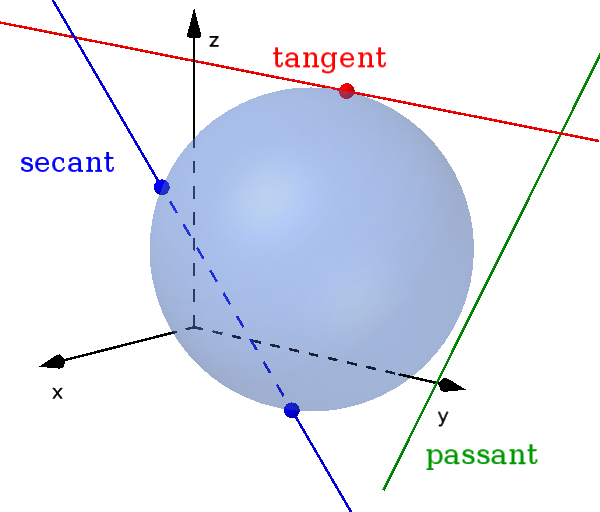Math Circles and spheres Spheres and lines

# Spheres and lines

There are three possible relative positions for a sphere and a line in space.!

### Remember

• A passant is a straight line that has no point in common with the sphere.

• A tangent has exactly one point in common.

• A secant has two different points in common with the sphere.

A sphere and a line can therefore have one, two or no common point.

The individual coordinates are used in the equation of a sphere to calculate the intersection points.

i

### Method

1. Write out coordinates of $g$
2. Insert and solve equations in the equation of a sphere
3. Insert $r$ into the line to get intersection(s)

### Example

$g: \vec{x} = \begin{pmatrix} 5 \\ 6 \\ 5 \end{pmatrix} + r \cdot \begin{pmatrix} 3 \\ 2 \\ 2 \end{pmatrix}$

$k: (x+1)^2+(y-2)^2$ $+(z-1)^2=17$

1. #### Break $g$ into 3 equations

We replace $\vec{x}$ and write out the respective coordinates as our own equation.

$\begin{pmatrix} x \\ y \\ z \end{pmatrix} = \begin{pmatrix} 5 \\ 6 \\ 5 \end{pmatrix} + r \cdot \begin{pmatrix} 3 \\ 2 \\ 2 \end{pmatrix}$

1. $x=5+3r$
2. $y=6+2r$
3. $z=5+2r$
2. #### Insert coordinates

The equations are now used in the equation of a sphere for $x$, $y$ and $z$.

$(x+1)^2+(y-2)^2$ $+(z-1)^2=17$

$(5+3r+1)^2$ $+(6+2r-2)^2$ $+(5+2r-1)^2=17$

$(6+3r)^2$ $+(4+2r)^2$ $+(4+2r)^2=17$

Use binomial theorem to resolve parentheses

$36+36r+9r^2$ $+16+16r+4r^2$ $+16+16r+4r^2=17$

$17r^2+68r+68=17\quad|-17$

$17r^2+68r+51=0\quad|:17$

$r^2+4r+3=0$

$r_{1,2}=-\frac{p}2\pm\sqrt{(\frac{p}2)^2-q}$
$r_{1,2}=-2\pm\sqrt{2^2-3}$
$r_{1,2}=-2\pm1$

$r_{1}=-1$ and $r_{2}=-3$

3. #### Insert $r$

The two calculated $r$ are inserted into the equation of a line in order to obtain the intersection points.

$\vec{OS_1} = \begin{pmatrix} 5 \\ 6 \\ 5 \end{pmatrix} - 1 \cdot \begin{pmatrix} 3 \\ 2 \\ 2 \end{pmatrix}$ $=\begin{pmatrix} 2 \\ 4 \\ 3 \end{pmatrix}$

$\vec{OS_2} = \begin{pmatrix} 5 \\ 6 \\ 5 \end{pmatrix} - 3 \cdot \begin{pmatrix} 3 \\ 2 \\ 2 \end{pmatrix}$ $=\begin{pmatrix} -4 \\ 0 \\ -1 \end{pmatrix}$

It is a secant that intersects the sphere at $S_1(2|4|3)$ and $S_2(-4|0|-1)$.